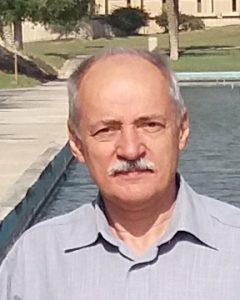# PRECUP Radu – Scientific researcher I

Corresponding member of the Romanian AcademyFields of interest/specialization: Nonlinear analysis; differential equations; partial differential equations; mathematical modelling.

Important results:

1. Homotopy and continuation results of Leray-Schauder and Granas type with applications to nonlinear boundary value problems.
2. Contributions to the vector approach of systems of equations.
3. Development of the critical point theory in conical shells and applications to existence, localization and multiplicity of positive solutions.
4. Discovering the role of Harnack type inequalities for the application of Krasnoselskii’s technique for the localization of solutions.
5. Contributions to the modelling and analysis of some biological processes.

Curriculum Vitae: Precup_CV